Next: 2.3.3 Transport-Independent Surface Reactions Up: 2.3 Surface Kinetics Previous: 2.3.1 Linear Surface Reactions

## 2.3.2 Non-Linear Surface Reactions

In general, the surface velocity (2.25) is not a linear function. As an example, the sticking probability in (2.31) can depend on the local arriving particle flux, if higher order surface kinetics are assumed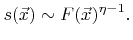(2.32)

Here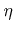is the order of the reaction. Obviously, in the case of non-linear reaction kinetics (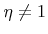) the sticking coefficient depends on the flux.

Other examples, where non-linear surface reactions need to be incorporated, are processes with ion-enhanced etching. There physical (ions) and chemical (neutrals) components act in a synergistic manner, so that the etch rate is larger than that obtained by summing up their individual contributions.

The Langmuir adsorption model is able to capture this behavior . The idea is to assume an absorbed state of byproducts. The fraction of surface sites covered by these byproducts is called the surface coverage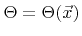. The etch rate is then composed of three contributions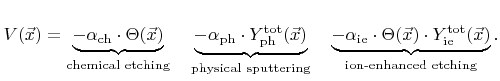(2.33)

The first term corresponds to chemical etching which is proportional to the coverage, the second term represents physical sputtering with the total sputter rate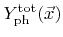, and the last term is due to ion-enhanced etching, which is proportional to the coverage and the total ion-enhanced etching rate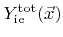. The total yields are both calculated using a weight function similar to that used in (2.27). Two different total yields are introduced, since in the general case the physical sputter yield and the ion-enhanced etching yield are not equal. The constants,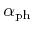, and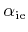in (2.33) are model parameters.

For the coverage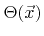a balanced equation can be set up as follows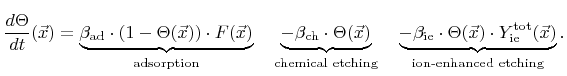(2.34)

The first term describes the adsorption of chemical components, which is proportional to the total arriving flux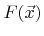of neutrals and the fraction of empty surface sites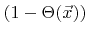. The second and the third term are losses due to chemical and ion-enhanced etching, respectively, which are both proportional to the coverage. The constants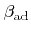,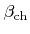, and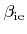are again model parameters.

A common approach is to assume that the coverage is always in a steady state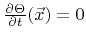. Therefore, the coverage can be explicitly expressed as a function of the ratesand,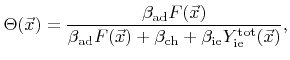(2.35)

and can be plugged into (2.33). The result is a non-linear function of the rates for the surface velocity.Next: 2.3.3 Transport-Independent Surface Reactions Up: 2.3 Surface Kinetics Previous: 2.3.1 Linear Surface Reactions

Otmar Ertl: Numerical Methods for Topography Simulation